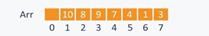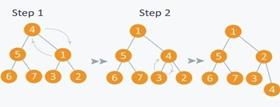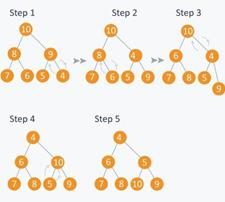# 8.3: Activity 3 - Heaps

## Introduction

A heap is a specific tree based data structure in which all the nodes of tree are in a specific order. Suppose that X is a parent node of Y, then the value of X follows some specific order with respect to value of Y and the same order will be followed across the tree. The maximum number of children of a node in the heap depends on the type of heap. However, a binary heap is the more commonly used heap type, in which there are at most 2 children of a node.

## Activity Details

In binary heap, if the heap is a complete binary tree with N nodes, then it has smallest possible height which is log 2 N. For example, from Figure $$\PageIndex{1}$$, observe a particular sequence that each node has greater value than any of its children. Suppose there are N Jobs in a queue to be done, and each job has its own priority. The job with maximum priority will get completed first than others. At each instant we are completing a job with maximum priority and at the same time we are also interested in inserting a new job in the queue with its own priority.Figure $$\PageIndex{1}$$: A binary heap

So at each instant we have to check for the job with maximum priority to complete it and also insert if there is a new job. This task can be very easily executed using a heap by considering N jobs as N nodes of the tree.

We can use an array to store the nodes of the heap tree. Consider a heap depicted in Figure $$\PageIndex{2}$$ and suppose that there are 7 elements with values {6, 4, 5, 3, 2, 0, 1}. We can use an array to simulate a tree in the following way: If we are storing one element at index, i, in array Arr, then its parent will be stored at index i/2 (unless it’s a root, since a root has no parent) and can be access by Arr[ i/2 ], and its left child can be accessed by Arr[ 2 * i ] and its right child can be accessed by Arr[ 2 * i +1 ]. The index of root in an array is 1.Figure $$\PageIndex{2}$$: Heap and Corresponding Array representation

There are two basic types of heap, max heap and min heap. In a max heap the value of parent node is always greater than or equal to the value of child node across the tree and the node with highest value is the root node of the tree. In a min heap the value of parent node is always less than or equal to the value of child node across the tree. Therefore the node with lowest value is the root node of tree.

### Max heap implementation

Suppose that you are given heap elements which are stored in array Arr. We can convert the array Arr into a heap structure as follows: Pick a node in the array, check if the left sub-tree and the right sub-tree are max heaps, among themselves and the node itself is a max heap (i.e., its value should be greater than all the child nodes). To perform this operation we define a function max_heapify that maintain the property of max heap (i.e. each element value should be greater than or equal to any of its child and smaller than or equal to its parent) as presented below:

void max_heapify (int Arr[ ], int i, int N)
{   int left  = 2*i             //left child
int right = 2*i +1          //right child
if(left<= N and Arr[left] > Arr[i] )
largest = left;
else
largest = i;
if(right <= N and Arr[right] > Arr[largest] )
largest = right;
if(largest != i )
{ swap (Ar[i] , Arr[largest]);
max_heapify (Arr, largest,N); }


The function max_heapify has time Complexity of O( log N ).

Now consider the example given in Figure $$\PageIndex{3}$$ depicts the max_heapify tree transformation steps. Initially 1st node (root node) is violating property of max-heap as it has smaller value than its children, so we are performing max_heapify function on this node with value 4. Since 8 is greater than 4, then 8 is swapped with 4 and max_heapify is performed again on 4, but on different position. Now in step 2, 6 is greater than 4, so 4 is swapped with 6 and we obtain a max heap, as now 4 is a leaf node, so further call to max_heapify will not create any effect on the heap. Therefore we can correct a violated max heap property by using max_heapify function.Figure $$\PageIndex{3}$$: Transformation steps to max heap

Now consider the heap property which states:

An N element heap stored in an array has leaves indexed by N/2+1, N/2+2 , N/2+3 …. up to N.

Figure $$\PageIndex{4}$$ demonstrates this heap property which is the heap derived from Figure $$\PageIndex{3}$$ whose elements have values {8, 7, 6, 3, 2, 4, 5}. Observe that the elements 3, 2, 4, 5 are indexed by N/2 +1 (i.e 4), N/2+2 (i.e 5 ) and N/2+3 (i.e 6) and N/2+4 (i.e 7) respectively.Figure $$\PageIndex{4}$$: Array indices for heap leaf elements

We can build a max heap out of the array elements. That is, suppose that we have N elements stored in the array Arr indexed from 1 to N, and possibly not following the property of a max heap. We use the max-heapify function to make a max heap out of the array. Based on the property stated above, we have that the elements from Arr[ N/2+1 ] to Arr[ N ] as leaf nodes, and each node is a 1 element heap. The max_heapify function can be used in a bottom up manner on remaining nodes, to cover each node of the heap tree. The code presented below captures these concepts to build up a max heap from a given array:

void build_maxheap (int Arr[ ])
{ for(int i = N/2 ; i >= 1 ; i-- )
{ max_heapify (Arr, i) ; }


Note that the time complexity for the build_maxheap function is O(N), since max_heapify function has complexity log N and the build_maxheap functions runs for only N/2 times.Figure $$\PageIndex{5}$$: An array Arr

Now we demonstrate the max_heap function function using a 7 elements array Arr, shown in Figure $$\PageIndex{5}$$. Here N = 7, so starting from node having index N/2 = 3, (i.e., with value 3 in Figure $$\PageIndex{5}$$), call max_heapify from index N/2 to 1. Figure $$\PageIndex{6}$$ depicts the build steps in which:

During step 1, in max_heapify(Arr, 3), since 10 is greater than 3, 3 and 10 are swapped and further call to max_heap(Arr, 7) will have no effect as 3 is a leaf node.Figure $$\PageIndex{6}$$: Tracing Max heap creation

During step 2, calling max_heapify(Arr, 2), sees node indexed with 2 has value 4, thus 4 is swapped with 8 and further call to max_heap(Arr, 5) will have no effect, since 4 is a leaf node.

During step 3, calling max_heapify(Arr, 1), sees node indexed with 1 has value 1, hence 1 is swapped with 10.

Step 4 is a subpart of step 3, as after swapping 1 with 10, again a recursive call of max_heapify(Arr, 3) will be performed, and 1 will be swapped with 9. Now further call to max_ heapify(Arr, 7) will have no effect, as 1 is a leaf node.

In step 5, we finally get a max- heap and the elements in the array Arr will be as shown in Figure $$\PageIndex{7}$$.Figure $$\PageIndex{7}$$: Resulting Max-heap array representation

The second heap type, min heap, has the value of parent node always less than or equal to the value of child node across the tree. Therefore the node with lowest value is the root node as depicted in Figure $$\PageIndex{8}$$. This figure also captures the fact that a node has a smaller value than the values of its children.Figure $$\PageIndex{8}$$: A min-heap

In a mini heap we can perform similar operations as in the max heap. Below we present a function min_heapify which maintains the min heap property:

void min_heapify (int Arr[ ] , int i, int N)
{ int left = 2*i;
int right = 2*i+1;
int smallest;
if(left <= N and Arr[left] < Arr[ i ] )
smallest = left;
else
smallest = i;
if(right <= N and Arr[right] < Arr[smallest] )
smallest = right;
if(smallest != i)
{ swap (Arr[ i ], Arr[ smallest ]);
min_heapify (Arr, smallest,N); }


Note that as in the max_heapify function, the time complexity of the min_heapify function is O (log N).

We demonstrate the above function by transforming the array Arr = {4, 5, 1, 6, 7, 3, 2}. Note from Figure $$\PageIndex{9}$$, that the element at index 1 is violating the property of min -heap, so performing min_heapify(Arr, 1) preserves the min-heap property.Figure $$\PageIndex{9}$$: Transforming to a min–heap

Apply the min_heapify function on remaining nodes other than leaves (since a leaf node is a 1 element heap), as defined by function build_minheap below to obtain to a min heap.

void build_minheap (int Arr[ ])
{   for( int i = N/2 ; i >= 1 ; i--)
min_heapify (Arr, i);  }


Note that as for the build_maxheap function the time complexity for the build_minheap function is O( N ).

We demonstrate the build_minheap function by transforming the elements in array {10, 8, 9, 7, 6, 5, 4}. To do so, need to run min_heapify on nodes indexed from N/2 to 1, where the node indexed at N/2 has value 9. The build_minheanp repeats this through a number of steps to obtain a min_heap as depicted in Figure $$\PageIndex{10}$$.Figure $$\PageIndex{10}$$: Building min-heap from array

## Conclusion

In this activity we presented heap data structure. We discussed two types of heap, max heap and min heap, along with the key operation of building a specified heap from an array. Heaps are considered as partially ordered tree, since the nodes of tree do not follow any order with their siblings (nodes on the same level). They are mainly used when setting priority to smallest or the largest node in the tree as we can extract these node very efficiently using heaps.

Assessment

1. A learner is required to undertake background reading, which is supported by lectures to explain various notions and to show the application of various techniques using examples. The coursework requires the students to solve exercises each week. Feedback for and help with this work is provided in the examples classes.